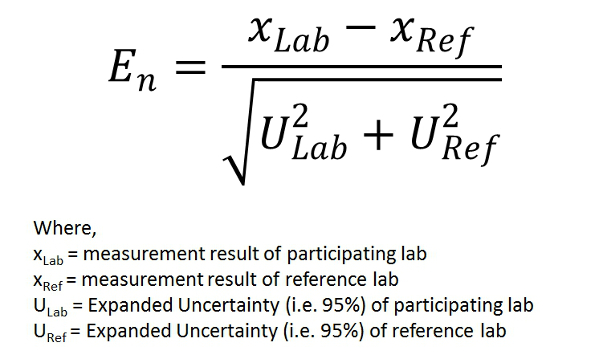# Statistical Analysis

Each artifact provided to participants has been calibrated by accredited laboratories whose accuracy and precision are well established.  Their measurement uncertainties are sufficiently small so as to minimize its contribution to results of the test.  These are identified as the reference value and reference uncertainty.

The results of our proficiency tests are expressed as a normalized error.  This is commonly referred to as an En (or E sub n).  The En is calculated using the formula below.

Once you submit your results for each test, we will have all data necessary to calculate and provide you the En for each artifact parameter using the formula below.The numerator of the equation is simply the difference between your value and the reference value.  The denominator for the equation is the square root of the sum of your uncertainty squared plus the reference laboratory uncertainty squared.

The En is a value that has no units.  An En of less than ± 1.0 is a pass while a value that is over 1.0 is an outlier.

You will be provided a preliminary report that will let you know if there are any outliers within one hour of your submission of the data sheet.

Should there be outliers, you will have time to determine what went wrong, and do corrective action.  You can then submit corrected data prior to returning the items to us.

Obviously, if all readings are under the 1.0 limit, no further action is needed.  Just return the items to Proficiency Testing, Inc. and we can provide the scores to you in your final report.